﻿ 双头铺放轨迹后处理技术研究 Research on Post-Processing Technique for Double-Heads Automated Placement

Material Sciences
Vol.07 No.09(2017), Article ID:23204,8 pages
10.12677/MS.2017.79102

Research on Post-Processing Technique for Double-Heads Automated Placement

Xianfeng Wang, Tao Liang, Jun Xiao

R & D Center for Composites Industry Automation, NUAA, Nanjing Jiangsu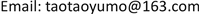Received: Dec. 3rd, 2017; accepted: Dec. 19th, 2017; published: Dec. 28th, 2017ABSTRACT

In order to improve the rate and kinematic dexterity of automated fiber placement system technology, a dual-robot collaborative placement of similar rotation component is studied, and the method of primary trajectory that fits the constraints of coordination is put forward. After determination of the matching scheme for the longitudinal feed of the robot, the AFP trajectory is paired by the Angle control, under the constraint of the robot platform’s full reachable workspace. At the same time, the track distribution scheme of the complete mould is obtained, and the information generated by CAD software is transformed into the machining document of robot Angle. This paper proves that the proposed method meets the process requirements of the production of large class gyro member, which greatly improves the laying efficiency.

Keywords:Automated Fiber Placement, Dual-Robot, Similar Rotation Component, Reachable Workspace, Trajectory Distribution1. 引言

2. 双头铺放系统行程范围计算

2.1. 坐标系的建立及包络方程的求解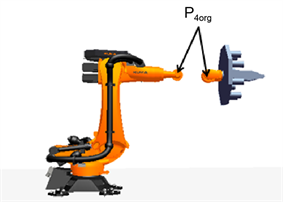(a) 位姿分离示意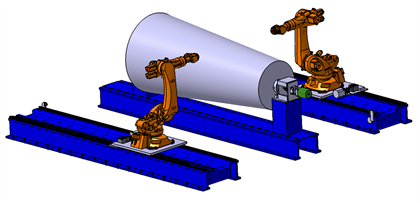(b) 拟采用机械结构

Figure 1. Structure schematic of dual-robot system

${G}_{{P}_{4}}=\left\{\begin{array}{l}{x}_{0}=\left({a}_{2}±\sqrt{{a}_{3}^{2}+{d}_{4}^{2}}\right){c}_{1}{c}_{2}+{a}_{1}{c}_{1}\\ {y}_{0}=\left({a}_{2}±\sqrt{{a}_{3}^{2}+{d}_{4}^{2}}\right){s}_{1}{c}_{2}+{a}_{1}{s}_{1}\\ {z}_{0}=\left({a}_{2}±\sqrt{{a}_{3}^{2}+{d}_{4}^{2}}\right){s}_{2}\end{array}$ (1)

${x}_{0}^{2}+{y}_{0}^{2}+{z}_{0}^{2}={\left({a}_{2}±\sqrt{{a}_{3}^{2}+{d}_{4}^{2}}\right)}^{2}+{a}_{1}^{2}+2\left({a}_{2}±\sqrt{{a}_{3}^{2}+{d}_{4}^{2}}\right){a}_{1}{c}_{2}$ (2)

${P}_{1}{P}_{2}:{\left(x-350\right)}^{2}+{\left(y-675\right)}^{2}={\left(1150+\sqrt{{41}^{2}+{1000}^{2}}\right)}^{2}$

${P}_{2}{P}_{3}:{\left(x-\left(350+1150\cdot \mathrm{cos}5\right)\right)}^{2}+{\left(y-\left(675+1150\cdot \mathrm{sin}5\right)\right)}^{2}={41}^{2}+{1000}^{2}$

${P}_{3}{P}_{4}:{\left(x-350\right)}^{2}+{\left(y-675\right)}^{2}={472}^{2}$

2.2. 铺丝头的干涉约束

2.3. 双机器人系统主轴位置的确定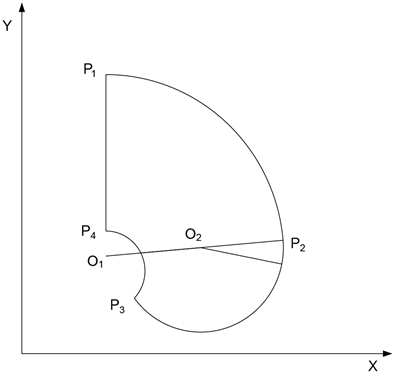Figure 3. A enveloping curve

3. 双头铺放轨迹分配策略

3.1. 两轨迹可铺放配对的判定

(1) 原始轨迹信息的参考坐标系为catia数模的坐标系，该坐标系与机器人基坐标系各轴指向可能不同。因此，在验证运算之前需要先对轨迹数据进行预处理，建立新的模具坐标系{B}(主轴坐标系)使得各轴指向与机器人基坐标系保持一致，坐标原点在模具回转轴线上。将包络模型转化到主轴坐标系下表示，简化位置关系的计算。设模具信息变换矩阵为 ${}_{I}^{B}T$ ，对于空间某点 ${\left(x,y,z\right)}^{I}$ ，该点在主轴坐标系下的坐标为：

${\left({x}_{r},{y}_{r},{z}_{r}\right)}^{B}{=}_{I}^{B}T{\left(x,y,z\right)}^{I}$ (3)

(2) 对轨迹信息中的所有数据点沿法向外延900 mm即可获得铺丝腕点位置信息。从轨迹数据中提取法向信息单位向量Normal $\left({x}_{n},{y}_{n},{z}_{n}\right)$ ，对于模具上某点 $\left({x}_{m},{y}_{m},{z}_{m}\right)$ ，获得外延后的腕点信息 $\left({x}_{w},{y}_{w},{z}_{w}\right)$ 为：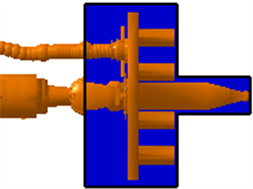(a) 铺丝头区域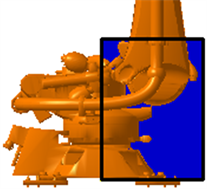(b) 底座区域

Figure 4. Interference area schematic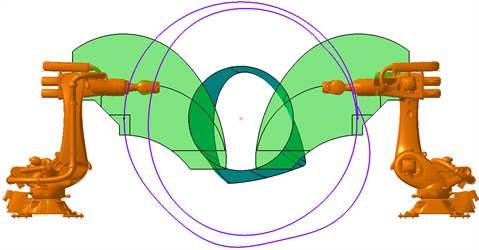Figure 5. Automatic placing of work space for molds

$\left\{\begin{array}{c}{x}_{w}={x}_{m}+900×{x}_{n}\\ {y}_{w}={y}_{m}+900×{y}_{n}\\ {z}_{w}={z}_{m}+900×{z}_{n}\end{array}$ (4)

(3) 对每一条轨迹中的腕点数据沿轴向即z方向提取特征点。定义提取方法，一是为轨迹调度合法性提供判断依据，二是为初始解的生成服务。出于简化计算考虑，对于轨迹1，从边界点a1开始逐点判断下一个点沿z与a1的差值是否大于某一个设定的值，记为列宽 $\xi$ 。如果大于 $\xi$ ，将此点记为a2，如此类推，将所有的特征点都提取出来。

(4) 对于不同两条轨迹中的两个特征点T1i、T2i沿法向延伸处理得到控制ki、mi，将其绕主轴旋转分别与两侧包络曲线上下限相交得到ki'、mi'、ki''、mi''。如图6所示，O点为模具主轴中心点。

3.2. 轨迹配对的优选

${p}_{i}=±k+W,1\le i\le m,$ (5)

4. 实例验证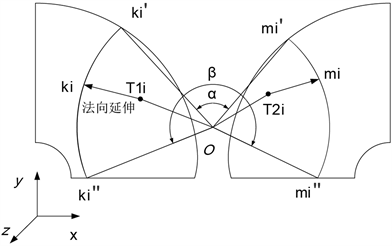Figure 6. The schematic diagram of Angle interval determines

5. 结论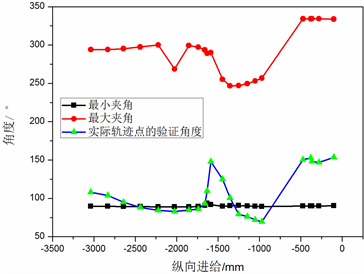(a)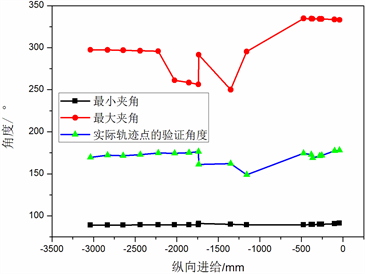(b)

Figure 8. Partial trajectory verification results

“高档数控机床与基础制造装备”科技重大专项：2016ZX04002-001-07。

Research on Post-Processing Technique for Double-Heads Automated Placement[J]. 材料科学, 2017, 07(09): 783-790. http://dx.doi.org/10.12677/MS.2017.79102

1. 1. Fei, Y.Q., Ding, F.Q. and Zhao, X.F. (2004) Collision-Free Motion Planing of Dual-Arm Reconfigurable Robots. Robotics and Computer-Integrated Manufacturing, 20, 351-357.
https://doi.org/10.1016/j.rcim.2004.01.002

2. 2. Chiddarwar, S.S. and Babu, N.R. (2009) Dynamic Priority Al-location for Conflict Free Coordinated Manipulation of Multiple Agents. Proceedings of IEEE International Conference on Automation Science and Engineering, IEEE, New York, 549-554.

3. 3. 甘亚辉, 戴宪中. 多机械臂协调控制研究综述[J]. 控制与决策, 2013, 28(3): 321-333.

4. 4. Kosuge, K., Ishikawa, J., Furuta, K., et al. (1990) Control of Sigle-Master Multi-Slave Manipulator System Using VIM. IEEM International Conference on Robotics and Automation, IEEE, Piscataway, 1172-1177.

5. 5. Zhu, W.H. (2005) On Adaptive Synchronization Control of Coordinated Multirobots with Flexible/Rigid Constraints. IEEE Transactions on Robotics, 21.

6. 6. 张鹏程, 张铁. 基于包络法六自由度工业机器人工作空间的分析[J]. 机械设计与制造, 2010(10): 164-166.

7. 7. 肖军, 李勇, 文立伟, 等. 树脂基复合材料自动铺放技术进展[J]. 中国材料进展, 2009, 28(6): 28-32.

8. 8. 梁宪珠, 张铖. 浅谈降低国内航空复合材料结构成本的途径[J]. 航空制造技术, 2011(3): 40-44.

9. 9. 富宏亚, 韩振宇, 路华. 纤维缠绕/铺带/铺丝成型设备的发展状况[J]. 航空制造技术, 2009(22): 43-46.

10. 10. 马香峰. 机器人机构学[M]. 北京: 机械工业出版社, 1991.

11. 11. 曹毅, 李秀娟, 宁祎, 杨冠英. 三维机器人工作空间及几何误差分析[J]. 机械科学与技术, 2006, 25(12): 1458-1462.

12. 12. 朱晋生, 王卓, 欧峰. 先进复合材料在航空航天领域的应用[J]. 新技术工艺, 2012(9): 76-79.

13. 13. Preffer, L.E. (1994) The Design and Control of a Two-Armed, Cooperating, Flexible-Drivetrain Robot System. Stanford University.

14. 14. 高为炳. 多机器人系统的动力学与协调控制[J]. 控制与决策, 1992, 7(3): 161-168.

15. 15. 陈国良, 黄心汉, 王敏. 双机器人协调完成复杂边缘跟踪的运动规划[J]. 电气自动化, 2006, 28(5): 253-257.

16. 16. 杜兆才, 邹方. 多机器人协调操作系统实现飞机大型部件对接的轨迹规划[J]. 航空制造技术, 2009(24): 88-91.

17. 17. Basile, F., Caccavale, F., Chiacchio, P., et al. (2012) Task-Oriented Motion Planning for Multi-Arm Robotic Systems. Robotics and Computer-Integranted Manufacturing, 28, 569-582.
https://doi.org/10.1016/j.rcim.2012.02.007

18. 18. Zhan, Q., He, Y.H. and Chen, M. (2003) Collision Avoidance of Cooperative Dual Reduant Manipulators. Chinese Journal of Aeronautics, 16, 117-122.
https://doi.org/10.1016/S1000-9361(11)60214-8